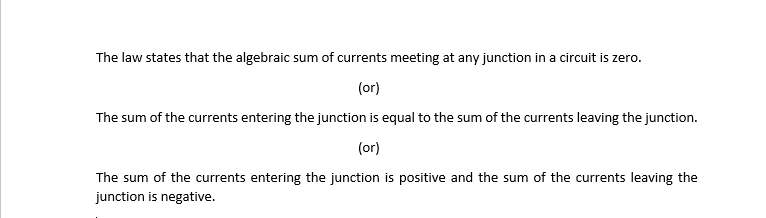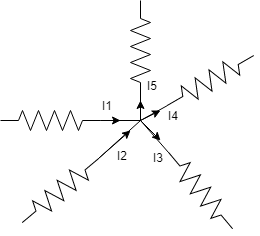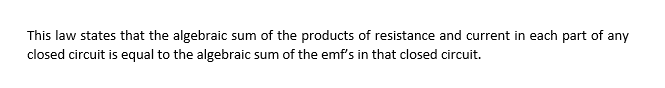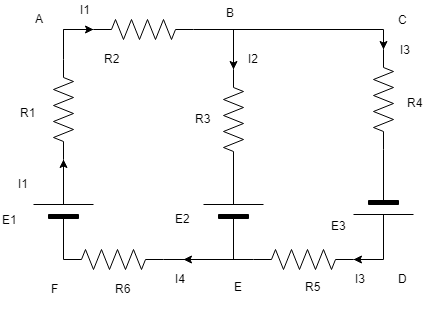# Kirchoff’s Current Law and Kirchoff’s Voltage Law | KVL and KCL

In this post, a clear explanation on KVL and KCL has been provided. Kirchoff’s Current Law and Kirchoff’s Voltage Law can be applied only a closed path or loop.

## Kvl & Kcl

Also, read: Equivalent Resistance | Calculation

### Kirchoff’s Current Law :In the above circuit, the I1, I2, I3, I4, and I5 are the currents flowing through each terminal. The  Current I1 and I2 flowing towards the junction and the currents I3, I4, and I5 flowing away from the circuit.

The KCL equation can be written as

I1 + I2 + (-I3) + (-I4) + (-I5) = 0

or

I1 + I2 = I3 + I4 + I5

Also, Read: Bcd -> Excess-3 Converter

### Kirchoff’s Voltage Law:Also, Read: Gray code -> Binary converter

The resistor in Series & the resistors in Parallel combination

While applying KVL, the clockwise direction is taken as a positive and the anti-clockwise direction is taken as a negative.

Here we have three sources, so in order to include all sources into the theorem, we need to apply KVL for the loops ABCDEFA and ABEFA.

For Loop ABCDEFA,

I1R1 + I1R2 + I3R4 + I3R5 + I4R6 + I1r1 + I3r3 = E1 + E3

For Loop ABEFA,

I1R1 + I1R2 + I2R3 + I4R6 + I2r2 + I1r1 = E1- E2

Also, Read: Excess-3 Converter -> bcd converter

## You may also Like: